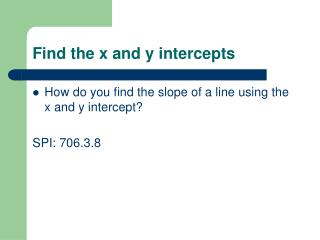Download PresentationFind the x and y intercepts

# Find the x and y intercepts

Download Presentation## Find the x and y intercepts

- - - - - - - - - - - - - - - - - - - - - - - - - - - E N D - - - - - - - - - - - - - - - - - - - - - - - - - - -
##### Presentation Transcript

1. Find the x and y intercepts • How do you find the slope of a line using the x and y intercept? SPI: 706.3.8

2. x- and y-Intercepts • The graphical concept of x- and y-intercepts is pretty simple. The x-intercepts are where the graph crosses the x-axis, and the y-intercepts are where the graph crosses the y-axis. • The problems start when we try to deal with intercepts algebraically.

3. To clarify the algebraic part, think again about the axes. When you were first introduced to the Cartesian plane, you were shown the regular number line from elementary school (the x-axis), and then shown how you could draw a perpendicular number line (the y-axis) through the zero point on the first number line. Take a closer look, and you'll see that the y-axis is also the line "x = 0". In the same way, the x-axis is also the line "y = 0".

4. Insert blank graphs here. (draw the x and y axes)

5. Then, algebraically, • an x-intercept is a point on the graph where y is zero • a y-intercept is a point on the graph where x is zero.

6. More specifically, • an x-intercept is a point in the equation where the y-value is zero, and • a y-intercept is a point in the equation where the x-value is zero.

7. The x-intercept of a line is the point at which the line crosses the x axis. ( i.e. where the y value equals 0 )x-intercept = ( x, 0 )The y-intercept of a line is the point at which the line crosses the y axis. ( i.e. where the x value equals 0 )y-intercept = ( 0, y )

8. Find the x and y intercepts of the equation 3x + 4y = 12. • To find the x-intercept, set y = 0 and solve for x. •  3x + 4( 0 ) = 12 3x + 0 = 12 3x = 12x = 12/3x = 4

9. Find the x and y intercepts of the equation 3x + 4y = 12. • To find the x-intercept, set y = 0 and solve for x. •  3x + 4( 0 ) = 12 3x + 0 = 12 3x = 12x = 12/3x = 4

10. Find the x and y intercepts of the equation 3x + 4y = 12. • To find the y-intercept, set x = 0 and solve for y. •   3( 0 ) + 4y = 12 0 + 4y = 12 4y = 12y = 12/4y = 3

11. Find the x and y intercepts of the equation 3x + 4y = 12. • To find the y-intercept, set x = 0 and solve for y. •   3( 0 ) + 4y = 12 0 + 4y = 12 4y = 12y = 12/4y = 3

12. Writing the Ordered Pair for the x and y intercepts • Once you have solved for both the x and y, you write your answers as ordered pairs. • So your x is going to be what you solved x to be and 0, because that is what you substituted for y in the equation. • Same thing for the y ordered pair: it is going to be 0 plus what you solved y to be.

13. Therefore, the x-intercept is ( 4, 0 ) and the y-interceptis ( 0, 3 ). • The graph of the line looks like this:

14.  Find the x and y intercepts of the equation 2x - 3y = -6. • To find the x-intercept, set y = 0 and solve for x. •   2x - 3( 0 ) = -6 2x - 0 = -6 2x = -6x = -6/2x = -3 • To find the y-intercept, set x = 0 and solve for y. •   2( 0 ) - 3y = -6 0 - 3y = -6 -3y = -6y = -6/(-3)y = 2

15. Therefore, the x-intercept is ( -3, 0 ) and the y-interceptis ( 0, 2 ). • The graph of the line looks like this:

16. Find the x and y intercepts of the equation ( -3/4 )x + 12y = 9. • To find the x-intercept, set y = 0 and solve for x. •   ( -3/4 )x + 12( 0 ) = 9 ( -3/4 )x + 0 = 9 ( -3/4 )x = 9x = 9/( -3/4 )x = -12 • To find the y-intercept, set x = 0 and solve for y. •   ( -3/4 )( 0 ) + 12y = 9 0 + 12y = 9 12y = 9y = 9/12y = 3/4

17. Therefore, the x-intercept is ( -12, 0 ) and the y-intercept is ( 0, 3/4 ). • The graph of the line looks like this:

18. Practice Pre-Algebra wb pg. 105